• turtle库随机走田字格（最小4×4每个路口 每个路口只能向上或者向右 每个路口方向随机 不能出界python
• 今天接着学习Python的turtle库的使用，我们用Python中的turtle库实现一些神奇走位的图案。 （一）神器走位图一 使用 turtle turtle.fd() 函数和 turtle.seth() 函数绘制螺旋状的正方形，正方形边长从 1 像素...今天接着学习Python的turtle库的使用，我们用Python中的turtle库实现一些神奇走位的图案。 （一）神器走位图一 使用 turtle 库的 turtle.fd() 函数和 turtle.seth() 函数绘制螺旋状的正方形，正方形边长从 1 像素开始，第一条边从 0 度方向开始。 1.整体代码 import turtle d = 0 k = 1 for j in range(10): for i in range(4): turtle.fd(k) d += 91 turtle.seth(d) k += 2 turtle.done() 2.效果图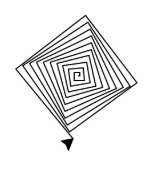（二）神器走位图二 使用turtle库的turtle.fd()函数和turtle.seth()函数绘制嵌套六角形，六角形边长从1像素开始，第一条边从0度方向开始，边长按照3个像素递增。 1.整体代码 import turtle edge = 6 d = 0 k = 1 for j in range(10): for i in range(edge): turtle.fd(k) d += 360/edge turtle.seth(d) k += 3 turtle.done() 2.效果图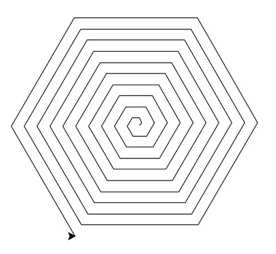（三）神器走位图三 使用 turtle 库的 turtle.forward() 函数和 turtle.left() 函数移动像素进行绘制。 1.整体代码 #coding=utf-8 import turtle import time
#同时设置pencolor=color1, fillcolor=color2 turtle.color(“red”, “yellow”)
turtle.begin_fill() for _ in range(50): turtle.forward(200) turtle.left(170) turtle.end_fill()
turtle.mainloop() 2.效果图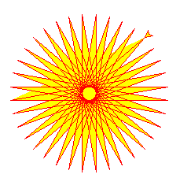以上就是本篇文章的全部内容，关注我带你看更多Python小技巧！ 文章部分内容源于网络，联系侵删* 文章参考源于http://h.zhimaruanjian.com/faq/54522.html
展开全文python
• 一、用random随机获取列表中的元素
一、用random随机获取列表中的元素
random中choice函数
import random
a = [1,2,3,4,5,6]
print(a[random.randint(0,5)])

等于
import random
a = [1,2,3,4,5,6]
print(random.choice(a))

均为随机数的产生
二、抬笔和落笔
turtle库默认为落笔状态 设置笔名为pen 抬笔:
pen.penup()

落笔：
pen.pendown()

完整代码：
import turtle
pen = turtle.Turtle()
pen.forward(200)
pen.left(90)
pen.penup()#抬笔
pen.forward(200)
pen.left(90)
pen.pendown()#落笔
pen.forward(200)
turtle.done()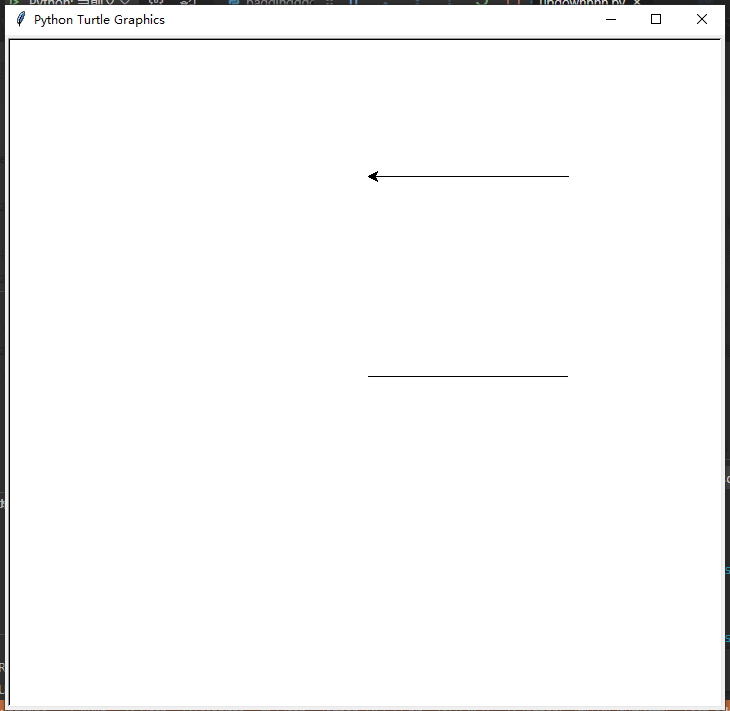三、背景图片的设置
准备一张格式为png的图片 其他格式不会辨认
import turtle
pen = turtle.Turtle()
#picture是我的文件夹
#alan.png是图片文件
#需要调用bgpic函数
turtle.bgpic("./picture/alan.png")
turtle.done()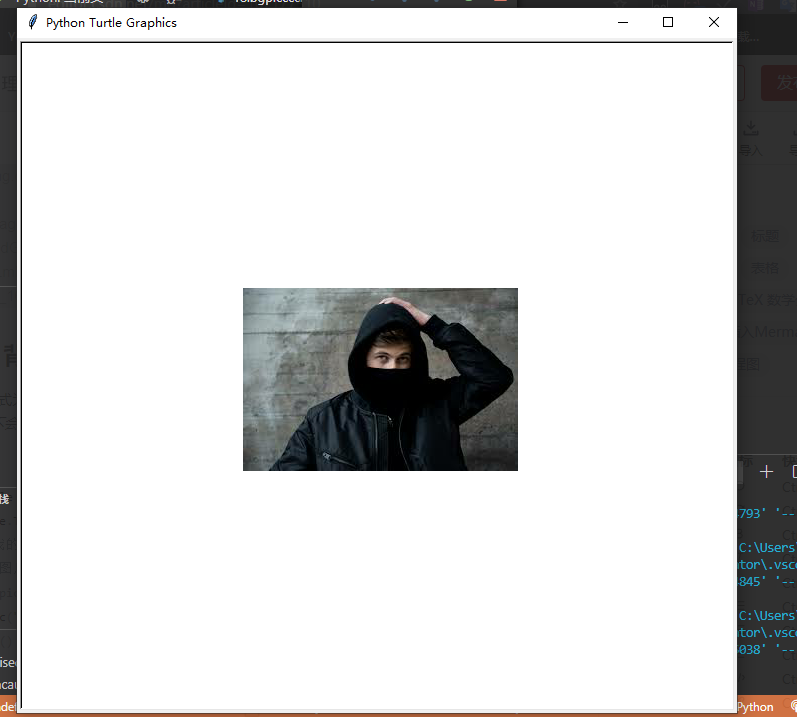四、窗口大小
需要调用setup函数 先看一下这个函数的参数
width#宽度
height#高度
startx#初始横坐标
starty#初始纵坐标

import turtle
pen = turtle.Turtle()
turtle.setup(width = 200 , height = 200 , startx = 0 , starty = 0)
turtle.done()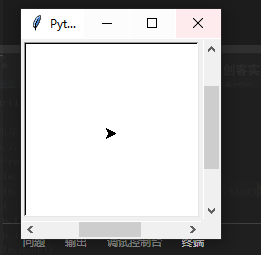可以发现，窗口变小了
展开全文python pycharm
• Turtle（海龟）1、用 random 随机取列表中的元素2、方法汇总2.1、画笔的抬笔和落笔2.2、画笔坐标的设置3、窗口大小和背景图片3.1、窗口大小的设置3.2、背景图片的设置3.3、背景图片和窗口大小相匹配 1、用 random ...


Turtle（海龟库）
1、用 random 随机取列表中的元素2、方法汇总2.1、画笔的抬笔和落笔2.2、画笔坐标的设置
3、窗口大小和背景图片3.1、窗口大小的设置3.2、背景图片的设置3.3、背景图片和窗口大小相匹配
4、五角星及多角星的画法

1、用 random 随机取列表中的元素
# 语法
a = [1, 2, 3, 4, 5]
# 创建一个列表
b = random.choice(a)
# 用 random.choice 从中随机取出一个元素放在变量 b 中

# 代码区
import turtle
import random

pen = turtle.Turtle()
# 创建一支画笔 pen
turtle.colormode(255)
# 先设置 colormode 选项
a = [1, 2, 3, 4, 5]
# 创建一个列表
b = random.choice(a)
# 用 random.choice 从中随机取出一个元素放在变量 b 中
pen.speed(0)
# 将画笔的速度设置为最快
for i in range(10):
pen.right(b * 5)
pen.color(random.randint(0, 255), random.randint(0, 255), random.randint(0, 255))
pen.circle(b * 100)

turtle.done()
# 让屏幕暂停

2、方法汇总
2.1、画笔的抬笔和落笔
# 语法
# 画笔抬笔 (不出现痕迹)
pen.penup()
# 画笔落笔 (出现痕迹)
pen.pendown()

# 代码区
import turtle

pen = turtle.Turtle()
# 创建一支画笔 pen
pen.forward(100)
# 画笔向右前进 100 个像素

# 画笔抬笔 (不出现痕迹)
pen.penup()
pen.right(90)
# 画笔右转 90°
pen.forward(100)
# 画笔前进 100 个像素

# 画笔落笔 (出现痕迹)
pen.pendown()
pen.right(90)
# 画笔右转 90°
pen.forward(100)
# 画笔前进 100 个像素

turtle.done()
# 让屏幕暂停

2.2、画笔坐标的设置
# 语法
pen.goto(0, 0)
# 使画笔回到原点

# 代码区
import turtle

pen = turtle.Turtle()
# 创建一支画笔 pen
for i in range(4):
pen.forward(100)
# 画笔前进 100 个像素
pen.goto(0, 0)
# 每次前进 100 个像素后回到原点
pen.right(90)
# 向右转 90°

turtle.done()
# 让屏幕暂停

3、窗口大小和背景图片
3.1、窗口大小的设置
# 语法
turtle.setup(width, height, startx, starty)
# width 是绘图区域宽度
# height 是绘图区域高度
# startx 和 starty 分别是距离左侧和上测屏幕的距离

# 代码区
import turtle

pen = turtle.Turtle()
# 创建一支画笔 pen

turtle.setup(width=800, height=800, startx=0, starty=0)
# 宽度和高度设置为 800 像素
# 离屏幕左侧和上测的距离设置为 0 像素

turtle.done()
# 让屏幕暂停

3.2、背景图片的设置
# 语法
turtle.bgpic("图片路径")

# 代码区
import turtle

pen = turtle.Turtle()
# 创建一支画笔 pen
turtle.bgpic("images\\love.gif")

turtle.done()
# 让屏幕暂停

3.3、背景图片和窗口大小相匹配
import turtle

pen = turtle.Turtle()
# 创建一支画笔 pen
turtle.bgpic("images\\love.gif")
# 图片像素为 500x500
turtle.setup(width=500, height=500, startx=0, starty=0)
# 宽度和高度设置为 500 像素
# 离屏幕左侧和上测的距离设置为 0 像素

turtle.done()
# 让屏幕暂停

4、五角星及多角星的画法
# 五角星
import turtle

pen = turtle.Turtle()
# 创建一支画笔 pen

for i in range(5):
pen.forward(100)
pen.right(180 - 180 / 5)

turtle.done()
# 让屏幕暂停

# 多角星
import turtle
import random

pen = turtle.Turtle()
# 创建一支画笔 pen
b = [5, 7, 9, 11, 13]
# 创建一个列表
a = random.choice(b)
# 用 random.choice 从中随机取出一个元素放在变量 b 中

for i in range(a):
pen.forward(100)
pen.right(180 - 180 / a)

turtle.done()
# 让屏幕暂停

展开全文python canvas random
• 编写一个Turtle程序显示一条随机路径，该路径从中心点开始在边界上的某点结束。或者在死点处结束（即该点被其他已经经过的4个点包围）。假设这个网格的大小是16*16。 （a）在死点结束的一条路径 （b）在边界点...
题目：
（a）在一个网格中的自我规避游走是一条从一点到另一点的路径，并且这条路径不会经过同一个点两次。自我规避游走在物理、化学和数学中有很多的应用。它可以用来模拟链状实体，例如溶剂和高分子聚合物。编写一个Turtle程序显示一条随机路径，该路径从中心点开始在边界上的某点结束。或者在死点处结束（即该点被其他已经经过的4个点包围）。假设这个网格的大小是16*16。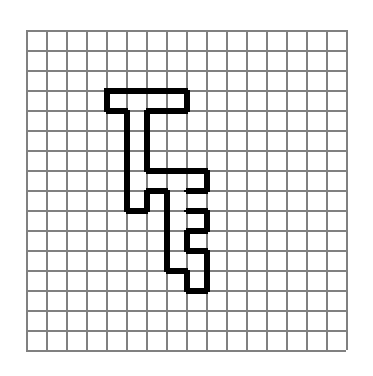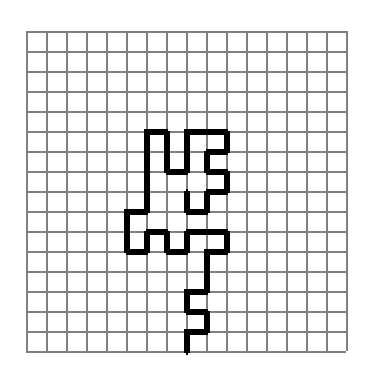（a）在死点结束的一条路径         （b）在边界点结束的一条路径
（b）再编写一个仿真程序来显示随着网格大小的扩大，路径在死点结束的概率将会提高。程序模拟网络大小从10变化到50。对于每一种网格大小，仿真10000次自我规避游走然后显示在死点结束的概率，输出如下所示。
For lattice of size 10, the probability of dead-end paths is 10.57%
For lattice of size 11, the probability of dead-end paths is 14.09%
……
For lattice of size 49, the probability of dead-end paths is 94.22%
For lattice of size 50, the probability of dead-end paths is 94.33%
示例代码：
import random
import turtle
count = 0#死点的计数
#判断是否走过
def Judge(xl,yl,listx,listy):
res=False
for i in range(len(listx)):
if xl==listx[i] and yl==listy[i]:#成对判断坐标是否已存在
res=True
return res
#判断是否死点
def Die(x,y,listx,listy):
x1=x+10
x2=x-10
y1=y-10
y2=y+10
Res=Judge(x1,y,listx,listy)&Judge(x2,y,listx,listy)&Judge(x,y1,listx,listy)&Judge(x,y2,listx,listy)
return Res
#地图可视化
def Map(size):
xs = -((size*10)//2)
turtle.pensize(1)
turtle.speed(10)
#纵坐标的线绘制
for y in range(-((size*10)//2),((size*10)//2)+1,10):
turtle.penup()
turtle.goto(xs,y)
turtle.pendown()
turtle.forward(size*10)
#横坐标线绘制
ys = ((size*10)//2)
turtle.right(90)
for x in range(-((size*10)//2),((size*10)//2)+1,10):
turtle.penup()
turtle.goto(x,ys)
turtle.pendown()
turtle.forward(size*10)
#路径绘制函数
def Draw(size):
global count
x = y = 0
listx=
listy=
#设定笔的属性
turtle.pensize(2)
turtle.speed(0)
turtle.color("red")
#模拟走动(是个方向等概率）
turtle.penup()
turtle.goto(0,0)
turtle.pendown()
while abs(x) < ((size*10)//2) and abs(y) < ((size*10)//2):
r = random.randint(0,3)#产生随机数，0右，1下，2左，3上表示是个方向
if Die(x,y,listx,listy):#判断死点
count+=1#计数
break
elif r == 0:#右
x += 10
if Judge(x,y,listx,listy):#判断是否为走过的点
x-=10 #是的话坐标不变
continue#终止本次循环
else:
listx.append(x)
listy.append(y)
turtle.forward(10)
elif r == 1:#下
y -= 10
if Judge(x,y,listx,listy):
y+=10
continue
else:
listx.append(x)
listy.append(y)
turtle.forward(10)
elif r == 2:#左
x -= 10
if Judge(x,y,listx,listy):
x+=10
continue
else:
listx.append(x)
listy.append(y)
turtle.forward(10)
elif r == 3:#上
y += 10
if Judge(x,y,listx,listy):
y-=10
continue
else:
listx.append(x)
listy.append(y)
turtle.forward(10)
#主程序部分
if __name__ == "__main__":
temp = input('请输入a,b来选择要解决的问题')
if temp=='a':
turtle.hideturtle()#隐藏画笔
Map(16)
Draw(16)
turtle.done()
elif temp=='b':
turtle.tracer(False)#隐藏动画效果
for i in range(10,51): #模拟地图规模变化
count=0#每次变化对死点计数器初始化
for j in range(0,10000):#10000次仿真训练
Draw(i)
turtle.reset()
print('For lattice of size ',i,', the probability of dead-end paths is ',count/100,'%')
else:
print('input error')

代码解释：
对于a部分，地图的可视化绘制的算法我将其封装为Map（）函数，通过两次for循环来根据指定距离和步长绘制网格地图。随机路径绘制的算法采用while循环的判断条件判断点的坐标的绝对值是否小于地图边长一半来确定点是否在地图内，如果在，通过随机种子的方法随机获取0到3中的某一数字，代表上下左右四个行进方向，之后首先通过if判断是否为死点，不是则继续用elif判断方向，之后通过嵌套的if判断该点是否走过，若走过，跳出此次循环，没有，将该点的x和y值存与列表中，并设置该方向的角度，沿该方向前进一格的距离，并将整个过程封装为Draw（）函数。判断死点和是否走过部分，我将其封装为Judge()和Die()两个函数,前者通过for循环成对遍历x和y的列表来判断即将要进入的坐标是否存在来判断是否走过该点，后者通过判断该点的上下左右四个点是否都成对存在于x和y的列表里来判断该点是否为死点。最后，调用这几个函数，并设定地图规模为16*16来完成a部分。对于b部分，实质上是对a部分算法的扩充，为了加快计算，设定隐藏动画效果，并取消运行地图可视化的Map（）函数，因为Map（）函数绘制的地图只是为了第一部分更直观的看到结果，其显示与否不影响路径绘制的算法。动态扩大地图范围的算法采用将地图边长作为参数传入Draw（）函数中，动态判断每次是否出边界。在主程序上首先输入a或者b，通过if判断要显示a还是b问题的结果，输入为a，则直接调用Map（）和Draw（）函数，并设定规模为16，输入为b，则使用for循环模拟地图规模从10到50的变化，通过嵌套的for循环执行10000模拟仿真游走，并根据要求按百分比输出死点的概率。
简要使用说明：
输入描述：输入a，启动解决a问题，输入b，启动解决b问题
输出描述：输入a时输出画图界面，显示16*16地图并按规则开始随机游走；输入b时，按要求输出从10到50地图规模每次10000次游走死点的概率。
输入输出的实例：
输入为a时的实例：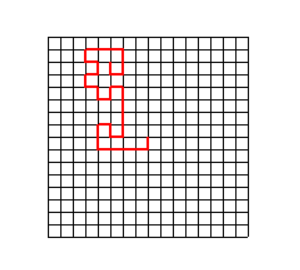输入为b时的实例：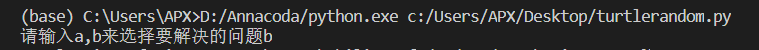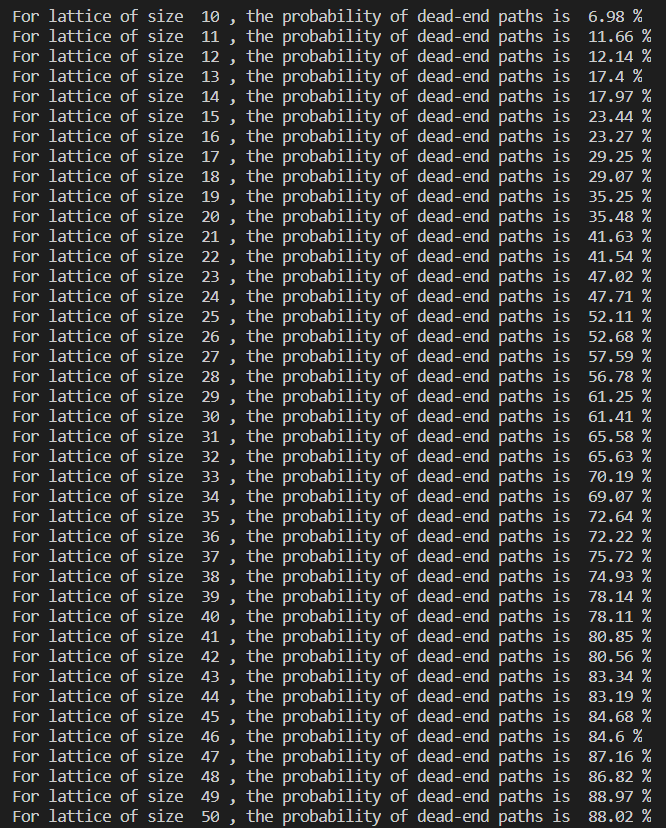展开全文python 算法
• 思路：用列表存储大小写字母、数字和下划线，用random随机产生一个下标对应一个字符然后画出图形。 import random a=['0','1','2','3','4','5','6','7','8','9','10','_'] for i in range(26): i+=65 r = chr(i) ...python
• from turtle import* pensize(2) pencolor("red") def sqaure(num): s = 600 penup() seth(-135) fd(400) speed(10) pendown() seth(90) fd(s) for i in range(1,num): seth(0) fd(s)python
• KaTeX parse error: Undefined control sequence: \首 at position 18: …olor{red}{大小写敏感\̲首̲字符非数字\不与保留相同} and elif import raise\color{red}{raise}raise global
• ## Python练习

千次阅读 2019-08-02 09:06:20
题目来自网络 Hello World的条件输出 获得用户输入的一个整数，参考该整数值，打印输出"Hello World"，要求：‪‬‪‬‪‬‪‬‪‬‮‬‪‬‫‬‪‬‪‬‪‬‪‬‪‬‮‬‭‬‪‬‪‬‪‬‪‬‪‬‪‬‮‬‪‬‪‬‪...
• ## 分享几个有趣的Python小项目

万次阅读 多人点赞 2020-05-04 14:42:48
一.经典的俄罗斯方块 1.绑定功能 # 绑定功能 class App(Frame): def __init__(self,master): Frame.__init__(self) master.bind('<Up>',self.Up) master.bind('<Left>',self.Left) ...Python python
• 1) #三个门随机生成初始值，0为羊，1为车 if m_1 == 1: m_2, m_3 = 0, 0 else: m_2 = randint(0, 1) if m_2 == 1: m_3 = 0 else: m_3 = 1 n = randint(1, 3) if n == 1: if m_1 == 1: count_n += 1 else: count_y +...python pycharm
• 2), (2, 2)]], # 反Z [[(0, 0), (0, 1), (1, 1), (1, 0)], [(0, 0), (0, 1), (1, 1), (1, 0)], [(0, 0), (0, 1), (1, 1), (1, 0)], [(0, 0), (0, 1), (1, 1), (1, 0)]], # [[(1, 0), (1, 1), (1, 2), (1, 3)], ...python
• Yertle the Turtle 剧中罗斯看的一本书——经典童话《乌龟大王亚特尔》，是美国著名童话作家聚斯的 作品。 第十集 Dick Clark 迪克•克拉克是每年主持纽约时代广场新年典礼的主持人，所以钱德勒说他是“迪克 •...测试 大数据 人工智能
• color = 'r') plt.plot(x, y2, color = 'r') plt.show() 2.Python绘制红色桃心 如果你觉得上面的代码颜色不够喜庆，接下来我们调用turtle库绘制动态红色的桃心。 from turtle import * #初始设置 setup(750,500) ...程序人生 程序员故事